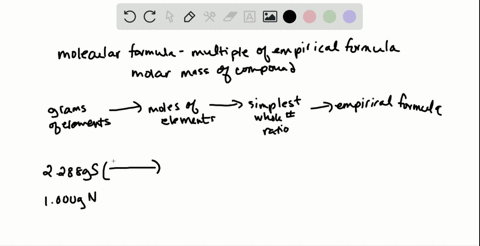🎉 The Study-to-Win Winning Ticket number has been announced! Go to your Tickets dashboard to see if you won! 🎉View Winning Ticket### The first sulfur-nitrogen compound was prepared i…

04:46University of Maine
Problem 97

# The mole is defined in terms of the carbon-12 atom. Use the definition to find (a) the mass in grams equal to 1 atomic mass unit; (b) the ratio of the gram to the atomic mass unit.

## Discussion

You must be signed in to discuss.

## Video Transcript

When we talk about measuring mass oven Adam, we can go back and forth between large and small skills by looking at either Adams or moles. Sometimes we use theater. Tomic Mass. Unit B A, M U for small skill. And then we typically use mass or grams so we can look at the relationship between these two units of small and large. The A M you were The atomic mass unit is based on the mass of a carbon 12 Adam. So one mole of carbon 12 has a mass of 12 grams. We also know that in one mole there are 6.2 times 10 to the 23rd Adams of carbon 12. So if we look at this relationship, we confined the mass of one atom of carbon 12. We're dividing 12 by ah for God rose number, which gives us 1.99 times 10 to the 23rd grams per adam at the molecular level. At the atomic level, one Adam of Carbon 12 has a mass in AM use of 12 atomic mass units or 12 AM use. If we know we have 1.99 times 10 to the minus 23 grams per kurban Adam, we can find the grams per atomic mass unit by dividing by 12 were 1.66 times 10 to the minus 24 grams per and new. So every one atomic mass unit is equivalent to 1.66 times 10 to the minus 24 grams. If we wanted to find out how many atomic mass units were in one gram, you simply take the reciprocal and it comes out to be a 6.2 times 10 to the 23rd AM use for every gram. So the ratio between grams and atomic mass units is Allah, God rose number.